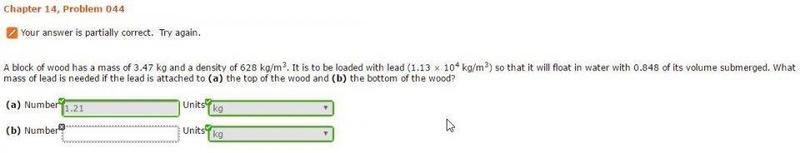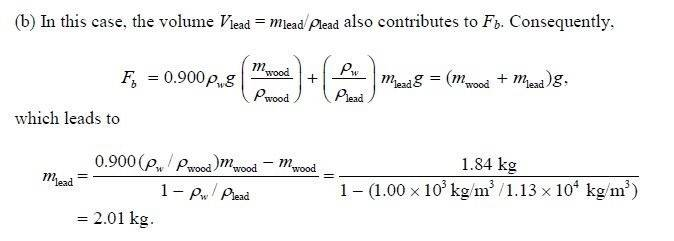# Density floating problem, wood and lead

1. Homework Statement
A block of wood has a mass of 3.47 kg and a density of 628 kg/m3. It is to be loaded with lead (1.13 × 104 kg/m3) so that it will float in water with 0.848 of its volume submerged. What mass of lead is needed if the lead is attached to (a) the top of the wood and (b) the bottom of the wood?alright so i have been working on this for a second and I am stuck on the second part...I found the solution to the book problem, it shows me how to do it but doesn't really flush out the steps. see below for the book problem solution.

## Homework Equations## The Attempt at a Solution

Sooo, with the above in mind, where did the (density of water)/(density of lead) come from and why is that extra M(lead) on the Fb side? I start with Fb=(Dw)(Vwood + Vlead)g, with Fb going to the above (Mwood+Mlead)g, g cancels....but I guess I am stuck there..

I tried going from that to solving for Mlead but it didn't really work out..I am checking my units and stuff and it looks ok....

in the example the 0.900 is equivalent to the 0.848, and i just treat it as a percent and multiply it in as shown. Are they using the density of water over the density of lead as a relative density??? I dont know..I am sure it's just a dumb mistake..

Thanks for any help.

Last edited:

Chestermiller
Mentor
What is the volume of the wood? What is the weight of the wood? What volume of wood is submerged? (This is the volume of water that the wood displaces)

Let M be the mass of lead. In terms of M, what is the weight of the lead? In terms of M, hat is the total weight of wood plus lead?

In the case of lead on top of the wood, there is no lead submerged. So what is the buoyant force of the water acting on the wood? How does this compare with the weight of the wood plus lead? You now have an equation for calculating the mass of lead M.

In the case that the lead is fastened underneath the wood, the entire block of lead is submerged. So, what is the volume of wood plus lead submerged (in terms of M)? What is the buoyant force of the water acting on the submerged wood and lead? How does this compare with the weight of the wood plus lead. You now have an equation for calculating the mass of lead M for this case.

chet

•Hey chet!

Yes, yes, I got all of that. I actually think I figured it out. I was thinking for (b) that the equation i needed to formulate was: